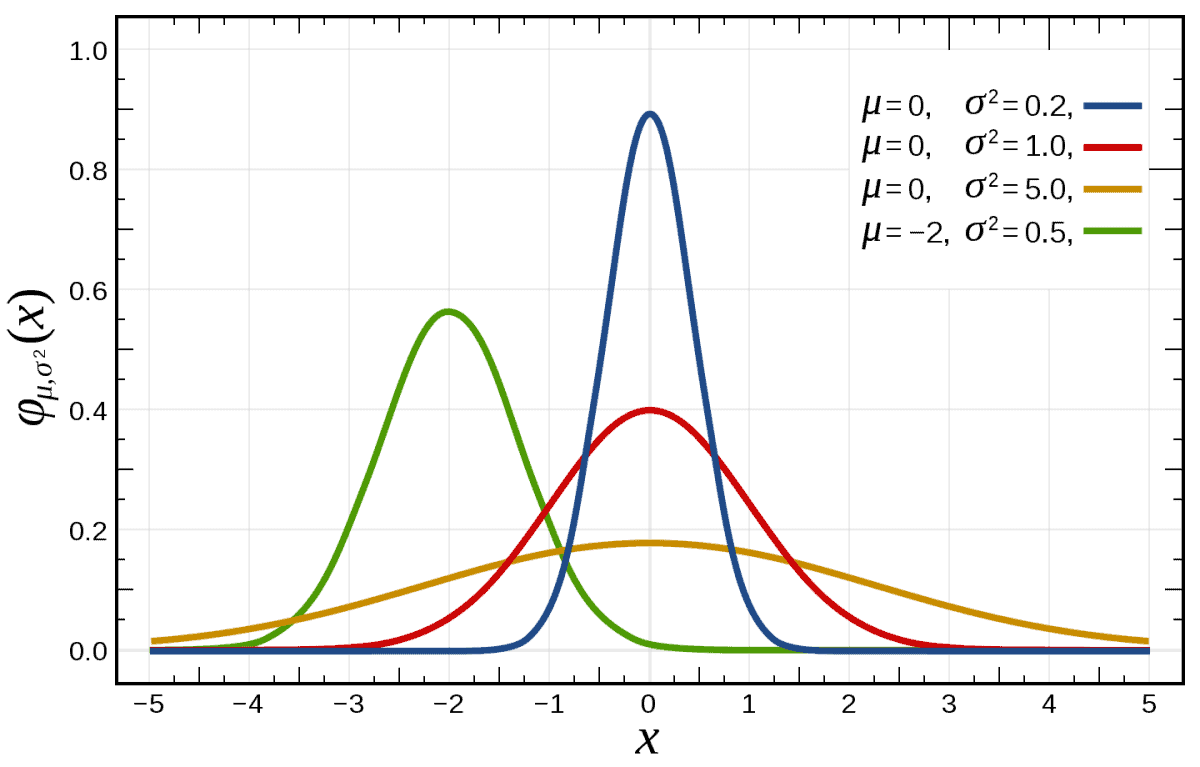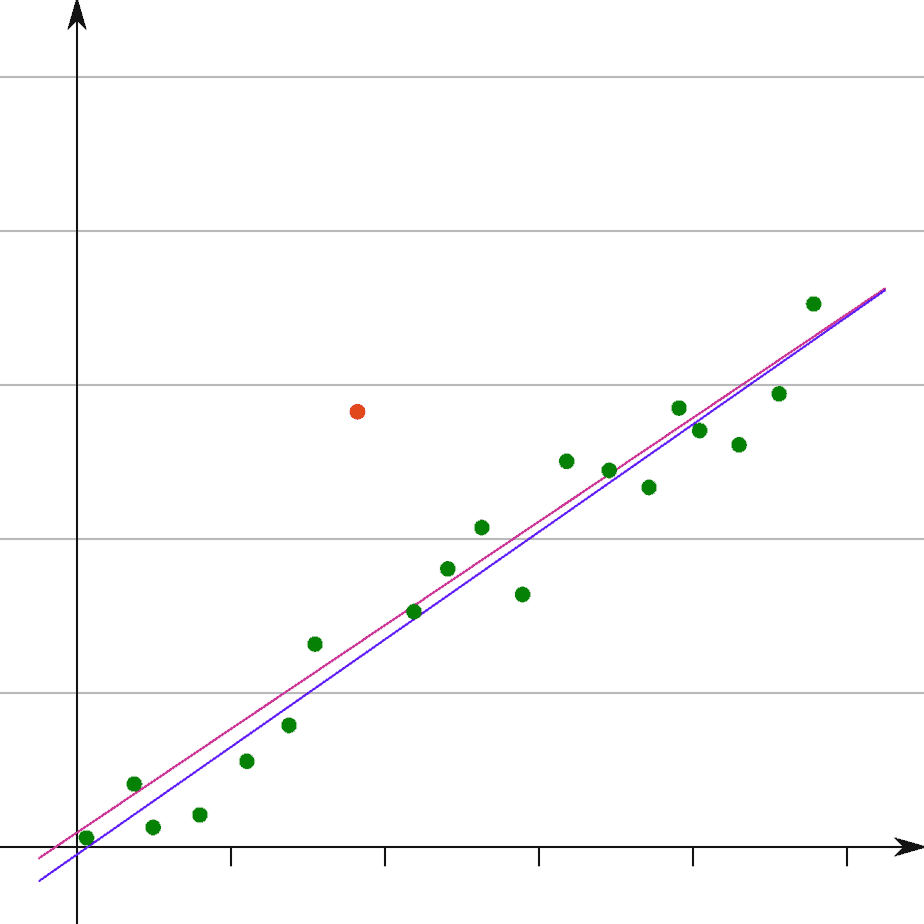# Variance (7 Common Questions Answered)

Variance is used in probability and statistics to help us find the standard deviation of a data set.  Knowing how to calculate variance is helpful, but it still leaves some questions about this statistic.

So, what do you need to know about variance?  Variance cannot be negative, but it can be zero if all points in the data set have the same value.  Variance can be less than standard deviation if it is between 0 and 1.  In some cases, variance can be larger than both the mean and range of a data set.  Finally, variance is affected by outliers.

Of course, there are very specific cases to pay attention to when looking at questions about variance.

In this article, we’ll answer 7 common questions about variance.  Along the way, we’ll see how variance is related to mean, range, and outliers in a data set.

Let’s get started.

## 7 Common Questions About Variance

After you learn how to calculate variance and what it means (it is related to the spread of a data set!), it is helpful to know the answers to some common questions that pop up.Variance tells us about how spread out the values in a data set are. A high variance means the data is spread out far from the mean (or there is at least one outlier far away from the mean).

A common one is about the sign of variance, so we’ll start there.

### Can Variance Be Negative?

Variance cannot be negative, since it is defined as the expected value of a squared term – specifically:

• V = E(X – M)2

where X is a random variable, M is the mean (expected value) of X, and V is the variance of X.

So the variance of a data set is a sum of squared values (which are positive) weighted by probabilities (which are also positive).  To find the variance, we:

• 1. Find the mean of the data set: M = E(X).
• 2. Take each data point and subtract the mean:calculateXi­ – M for each I (every point in the data set).
• 3. Square each difference: (Xi­ – M)2.
• 4. Multiply each squared difference by the probability of Xi (often, the probabilities are all assumed to be 1/N, where N is the number of data points.)
• 5. Add up all of the products from the last step.

Since each difference is a real number (not imaginary), the square of any difference will be nonnegative (that is, either positive or zero).  When we add up all of these squared differences, the sum will be nonnegative.

Most of the time, variance will be positive.  However, there is one special case where variance can be zero.

### Can Variance Be Zero?

Variance can be zero in the special case when all of the data points have the exact same value.  In that case, the mean has the same value as every point in the data set, and we have:

• Xi = K for all i (that is, every point in the data set has a value of K).
• M = E(X) = K (the mean is K, since every point in the data set has a value of K).
• Xi – M = 0 for all i (since Xi = K for all i)
• (Xi – M)2 = 0 for all i (since 02 = 0)

When we add up all of the squared differences (which are all zero), we get a value of zero for the variance.A uniform distribution has zero variance. Every point in the data set has the same value, which is also the value of the mean.

Note that this also means that the standard deviation is zero, since standard deviation is the square root of variance.

Based on this definition, there are some cases when variance is less than standard deviation.

### Can Variance Be Less Than Standard Deviation?

Variance can be less than standard deviation if the standard deviation is between 0 and 1 (equivalently, if the variance is between 0 and 1).

Remember that variance and standard deviation are connected by the equation

• V(X) = [S(X)]2  [variance is the standard deviation squared]

Or, equivalently:

• S(X) = [V(X)]1/2  [standard deviation is the square root of variance]

When 0 < S(X) < 1, we will get a smaller number when we square S(X).  The reason is that we are multiplying by a number smaller than 1, so we will get a smaller number for the product.

For example:

• 0.92 = 0.81 (0.81 < 0.9)
• 0.52 = 0.25 (0.25 < 0.5)
• 0.12 = 0.01 (0.01 < 0.1)

Just remember that standard deviation and variance have difference units.  Standard deviation is in linear units, while variance is in squared units.

### Can Variance Be Greater Than 1?

Variance can be greater than 1 in many cases.  In fact, if every squared difference of data point and mean is greater than 1, then the variance will be greater than 1.

Note that this also means the standard deviation will be greater than 1.  The reason is that if a number is greater than 1, its square root will also be greater than 1.

For example:

• 41/2 = 2  [both 4 and 2 are greater than 1]
• 2.251/2 = 1.5  [both 2.25 and 1.5 are greater than 1]
• 1.211/2 = 1.1  [both 1.21 and 1.1 are greater than 1]

### Can Variance Be Greater Than Mean?

Variance can be greater than mean (expected value) in some cases.  For example, when the mean of a data set is negative, the variance is guaranteed to be greater than the mean (since variance is nonnegative).

#### Example 1: Variance Greater Than Mean (Negative Mean)

Let’s consider the data set {-4, -3, -2, -1, 0}.

This data set has a mean of (-4 + -3 + -2 + -1 + 0) / 5 = -10 / 2 = -2.

The variance is 2.

Since 2 > -2, we have a variance that is greater than the mean.

However, it is still possible for variance to be greater than the mean, even when the mean is positive.

#### Example 2: Variance Greater Than Mean (Positive Mean)

Let’s consider the data set {-1, 0, 1, 2, 3}.

This data set has a mean of (-1 + 0 + 1 + 2 + 3) / 5 = 5/5 = 1.

The variance is 2.

Since 2 > 1, we have a variance that is greater than the mean.

However, there are cases when the variance can be less than the mean.

#### Example 3: Variance Less Than Mean (Zero Variance & Positive Mean)

Let’s consider the data set {2, 2, 2, 2, 2}.

The mean is 2, and the variance is zero.

Remember that if the mean is zero, then variance will be greater than mean unless all of the data points have the same value (in which case the variance is zero, as we saw in the previous example).

Remember that variance and mean have different units.  Mean is in linear units, while variance is in squared units.

### Can Variance Be Larger Than Range?

Variance can be larger than range (the difference between the highest and lowest values in a data set).  However, this is not always the case.

#### Example 1: Variance Larger Than Range

Let’s consider the data set {-100, -50, 0, 50, 100}.

The range is the difference between the maximum and minimum values in the data set:

• Range = Maximum – Minimum
• Range = 100 – (-100)
• Range = 200

The variance in this case is 5000 (it is large because the mean is zero and some data values are far away from the mean).

However, there are cases where variance can be less than the range.

#### Example 2: Variance Less Than Range

Let’s consider the data set {-1, -0.5, 0, 0.5, 1}.

The range is the difference between the maximum and minimum values in the data set:

• Range = Maximum – Minimum
• Range = 1 – (-1)
• Range = 2

The variance in this case is 0.5 (it is small because the mean is zero, the data values are close to the mean, and the differences are at most 1).

Remember that variance and range have different units.  Range is in linear units, while variance is in squared units.

### Is Variance Affected By Outliers?

Variance is affected by outliers.  An outlier changes the mean of a data set (either increasing or decreasing it by a large amount).An outlier (like the red dot above) are data points that are far outside of the expected range of values, or ones that lie far away from other data points. An outlier can have a drastic effect on both mean and variance.

The mean goes into the calculation of variance, as does the value of the outlier.  So, an outlier that is much greater than the other data points will raise the mean and also the variance.

Likewise, an outlier that is much less than the other data points will lower the mean and also the variance.

#### Example: Outliers Affect Variance & Mean

Let’s consider the data set {0, 1, 2, 3, 4}.

The mean is 2, and the variance is 2.

However, if we replace 4 with the outlier 99, we get the data set {0, 1, 2, 3, 99}.

The mean is now 21, and the variance is now 1522.

So variance is affected by outliers, and an extreme outlier can have a huge effect on variance (due to the squared differences involved in the calculation of variance).

## Conclusion

Now you know the answers to some common questions about variance.  You have also seen some examples that should help to illustrate the answers and make the concepts clear.